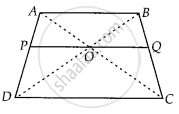# O is the point of intersection of the diagonals AC and BD of a trapezium ABCD with AB || DC. Through O, a line segment PQ is drawn parallel to AB meeting AD in P and BC in Q. Prove that PO = QO. - Mathematics

Sum

O is the point of intersection of the diagonals AC and BD of a trapezium ABCD with AB || DC. Through O, a line segment PQ is drawn parallel to AB meeting AD in P and BC in Q. Prove that PO = QO.

#### Solution

Given ABCD is a trapezium. Diagonals AC and BD are intersect at O.

PQ || AB || DC

To prove: PO = QOProof: In ∆ABD and ∆POD, PO || AB  .....[∵ PQ || AB]

∠D = ∠D   .....[Common angle]

∠ABD = ∠POD  .....[Corresponding angles]

∴ ∆ABD ~ ∆POD  ......[By AA similarity criterion]

Then, (OP)/(AB) = (PD)/(AD)  .......(i)

In ∆ABC and ∆OQC, OQ || AB

∠C = ∠C  ......[Common angle]

∠B AC = ∠QOC  ......[Corresponding angles]

∴ ∆ABC ~ ∆OQC  .....[By AA similarity criterion]

Then, (OQ)/(AB) = (QC)/(BC)  ......(ii)

Now, In ∆ADC, OP || DC

∴ (AP)/(PD) = (OA)/(OC) [By basic proportionality theorem]   .......(iii)

In ∆ABC, OQ || AB

∴ (BQ)/(QC) = (OQ)/(OC) [By basic proportionality theorem] ......(iv)

From equations (iii) and (iv), we get

(AP)/(PD) = (BQ)/(QC)

Adding 1 on both sides, we get

(AP)/(PD) + 1 = (BQ)/(QC) + 1

⇒ (AP + PD)/(PD) = (BQ + QC)/(QC)

⇒ (AD)/(PD) = (BC)/(QC)

⇒ (PD)/(AD) = (QC)/(BC)

⇒ (OP)/(AB) = (QC)/(BC)  ....[From equation (i)]

⇒ (OP)/(AB) = (OQ)/(AB)  .....[From equation (ii)]

⇒ OP = OQ

Hence proved

Concept: Basic Proportionality Theorem (Thales Theorem)
Is there an error in this question or solution?

#### APPEARS IN

NCERT Mathematics Exemplar Class 10
Chapter 6 Triangles
Exercise 6.4 | Q 15 | Page 75

Share